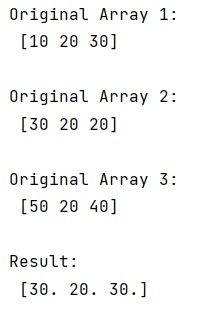# How to get the element-wise mean of a NumPy ndarray?

Given a NumPy ndarray, we have to get its element-wise mean.
Submitted by Pranit Sharma, on February 26, 2023

NumPy is an abbreviated form of Numerical Python. It is used for different types of scientific operations in python. Numpy is a vast library in python which is used for almost every kind of scientific or mathematical operation. It is itself an array which is a collection of various methods and functions for processing the arrays.

## Calculating the element-wise mean of a NumPy ndarray

Suppose that we are given three numpy ndarrays which contain some numerical values and we need to find the element-wise mean of these arrays simultaneously.

One of the methods for solving this problem is the vectorized sum of the ndarrays and dividing it by the total elements.

But, for this purpose, we can directly use the numpy.mean() method where we can pass a list containing all the arrays for which we want to calculate the mean.

Also, in pandas, pandas DataFrames have built-in operations to get column and row means.

Let us understand with the help of an example,

## Python code to calculate the element-wise mean of a NumPy ndarray

```# Import numpy
import numpy as np

# Creating three numpy arrays
arr1 = np.array([10, 20, 30])
arr2 = np.array([30, 20, 20])
arr3 = np.array([50, 20, 40])

# Display original arrays
print("Original Array 1:\n",arr1,"\n")
print("Original Array 2:\n",arr2,"\n")
print("Original Array 3:\n",arr3,"\n")

# Finding element wise mean of arrays
res = np.mean([arr1,arr2,arr3],axis=0)

# Display result
print("Result:\n",res,"\n")
```

Output:Languages: » C » C++ » C++ STL » Java » Data Structure » C#.Net » Android » Kotlin » SQL
Web Technologies: » PHP » Python » JavaScript » CSS » Ajax » Node.js » Web programming/HTML
Solved programs: » C » C++ » DS » Java » C#
Aptitude que. & ans.: » C » C++ » Java » DBMS
Interview que. & ans.: » C » Embedded C » Java » SEO » HR
CS Subjects: » CS Basics » O.S. » Networks » DBMS » Embedded Systems » Cloud Computing
» Machine learning » CS Organizations » Linux » DOS
More: » Articles » Puzzles » News/Updates